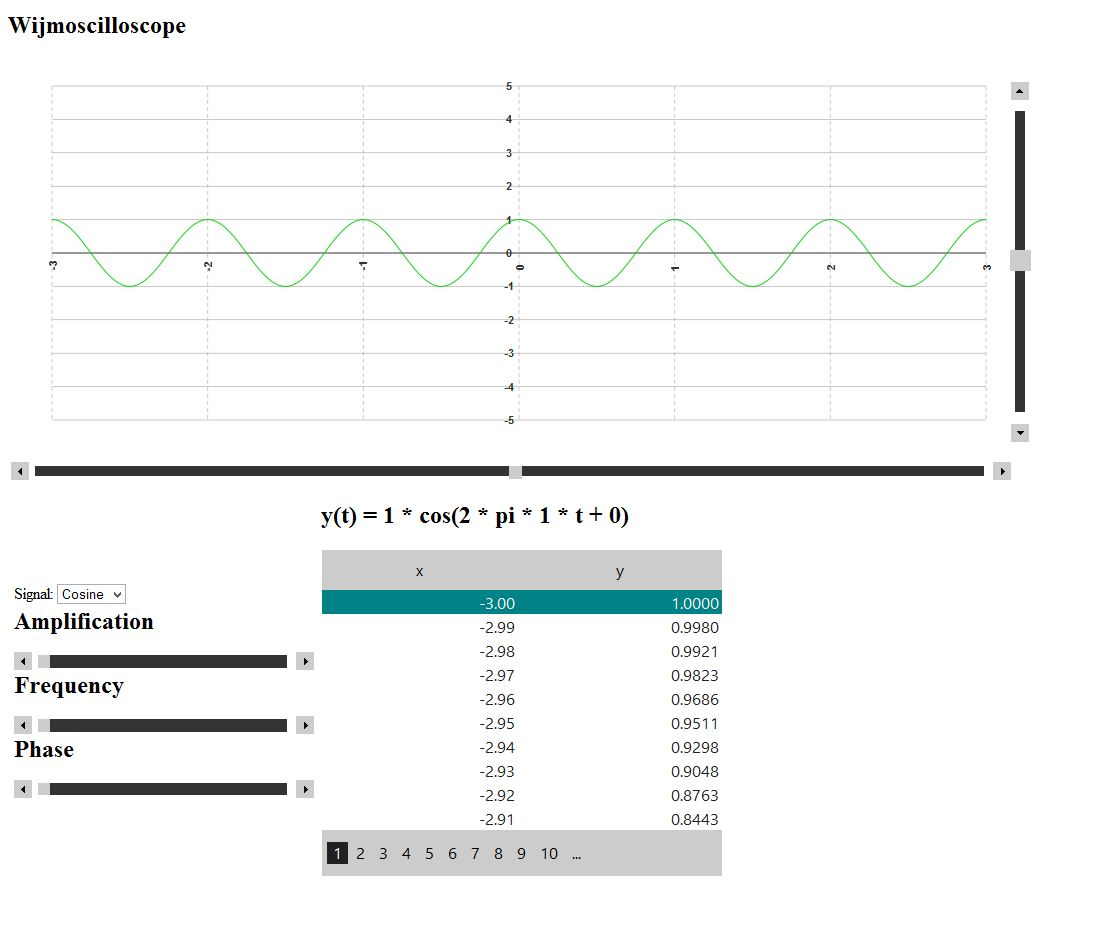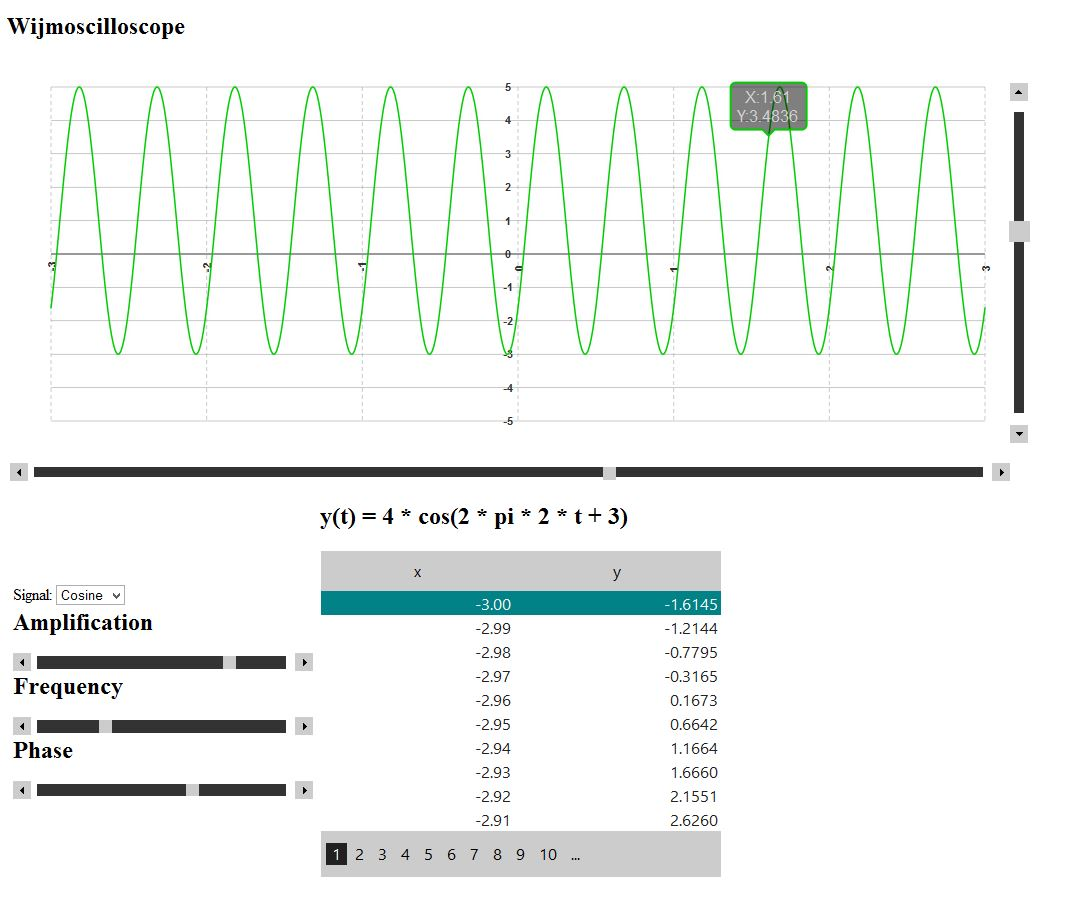﻿ Wijmoscilloscope: The Power of Knockout# Wijmoscilloscope: The Power of Knockout

Wijmo is a fantastic tool to make your data visible. Along with its partner Knockout, you can tie all of your information easily and elegantly. This got me to thinking, “What kind of data would I want to see easily?” Coming for an engineering background, I used pretty much everything under the sun (graphing calculators, websites, different software) to interpret signals. Probably the most useful tool of all was the oscilloscope. In the simplest explanation, the oscilloscope is used to graph, manipulate, and measure an input signal. For the purposes of this sample, I will keep the input signal simple and use the basic sine and cosine as functions of time: y(t) = A sin(2pi\f*t + _φ_) and y(t) = A cos(2pi\f*t + _φ_) where A = amplitude, f = frequency, and _φ_ is the phase. What do I want to see?

1. A plot of the input function.
2. Individual point information on the plot.
3. Information about each point in grid form.
4. The formula for the input function.

What do I want to do?

1. Manipulate the input signal by changing the amplitude, frequency, and phase.
2. Perform the oscilloscope feature of shifting the input signal horizontally and vertically on the plot.
3. Scroll over the plot of the wave and see individual (x,y) coordinates.

Using Wijmo and Knockout, all of this becomes possible, and most importantly, completely live. Let’s begin with the ViewModel: Using knockout observables, I am able to have a single integer value for each variable that will change about the input function and the plot.

`````` var self = this;

// declare observables that make up the object model
self.chartType = ko.observable("cos");
self.amp = ko.observable(1);
self.freq = ko.observable(1);
self.phase = ko.observable(0);
``````

Using knockout computed values, I am able to write function to return a live updated string of the function formula.

`````` // formula for current equation
self.formula = ko.computed(function () {
return "y(t) = " + self.amp() + " * " + self.chartType() + "(2 * pi * " + self.freq() + " * t + " + self.phase() + ")";
});
``````

Also using knockout computed values, I am able to create an array of plot points based on the input function. This list will be the common data for the chart and the grid, with no other manipulation necessary.

``````// compute data for both grid and list
self.list = ko.computed(function () {
var arr = [];

for (var x = -3; x<=3; x=x+.01){
if (self.chartType() == "cos") {
var y = self.amp() * Math.cos((6.28318 * self.freq() * x) +
y = Math.round(10000 * y) / 10000;
}
else {
var y = self.amp() * Math.sin((6.28318 * self.freq() * x) +
y = Math.round(10000 * y) / 10000;
}

// push the computed point into the chart data
x = Math.round(100*x)/100;
var point = new DataPoint({ x: x, y: y });
arr.push(point);
}
return arr;
});

``````

For the Model, I will create individual DataPoints using Knockout observables.

``````// datapoints that can be bound to both the chart and grid
function DataPoint(data) {
this.x = ko.observable(data.x);
this.y = ko.observable(data.y);
};
``````

In the \$(document).ready, I will bind the ViewModel, and set a few Wijmo decorators.

``````\$(document).ready(function () {

// create and bind the ViewModel
var vm = new viewModel();
ko.applyBindings(vm);

// wijmo Decorators
\$(":input[type='button']").button();
});
``````

Inside of the body, I will use the Wijchart to plot the data, the Wijgrid to show each point, Wijsliders to manipulate the input function variable values and adjust the chart horizontally and vertically, and an HTML dropdown list to toggle sine/cosine for the input function.

#### The Chart

Notice the dataSource for the chart is bound to the list. Inside of the SeriesList, I am able to bind the data to the X and Y values from the Model. Inside of the hint, I can also bind the content according to the point hovered over:

`````` <div id="wijlinechart" data-bind="wijlinechart: {
animation: {direction:'vertical'},
legend: {visible:false},
hint: {
content: function() {
return 'X:' +
this.x + '\\nY:' + this.y;
}
},
showChartLabels: false,
dataSource: list,
seriesList: [{
label: 'wave',
fitType: 'spline',
data: { x: { bind: 'x' }, y: { bind: 'y'} }
}],
axis: {
x: {
origin:0,
labels: { style: { rotation: -90 } },
annoMethod: 'valueLabels',
valueLabels: [-4,-3,-2,-1,0,1,2,3,4],
min: -3.14,
max: 3.14,
style: {'stroke-width': 2},
gridMajor: { visible: true},
},
y: {
max: 5,
min: -5,
autoMax: false,
autoMin: false,
style: {opacity: 1,'stroke-width': 6},
origin: 0,
}
}
}"
style="width:1000px;height:400px;"> </div>
``````

#### The Grid

The Grid is also bound to the same list as the chart with no changes needed.

``````<table id ="wijgrid" data-bind="wijgrid: {
allowPaging: true,
pageSize: 10,
data: list,
ensureColumnPxWidth: true,
columns: [
{ dataType: 'number', dataFormatString: 'n2' },
{ dataType: 'number', dataFormatString: 'n4' },
]
}"
style="width:400px;"></table>
``````

#### The Variable Sliders and Horizontal/Vertical Adjust

Each is set up the same. The Value of the slider will be bound to its knockout observable.

``````<div id ="phase" data-bind="wijslider: {
orientation: 'horizontal',
min: 0,
max: 5,
step: 1,
value:phase}",
style="width:300px;"></div>
``````

#### The Function

Using a simple heading, I can bind the text to the Knockout computed value.

``````<h2 data-bind="text: formula"></h2>
``````

That’s all of the good stuff. Now let’s take it for a spin!You can start moving the sliders to change the input function. Notice how the data in the formula, chart, and grid change instantly! Using the Knockout MVVM library along with Wijmo, you are able to create useful and interactive way to view your data with a relatively small amount of code.Wijmo with a Knockout backbone prevails again! Complete code can be found here.GrapeCity

GrapeCity Developer Tools